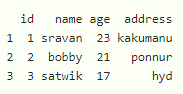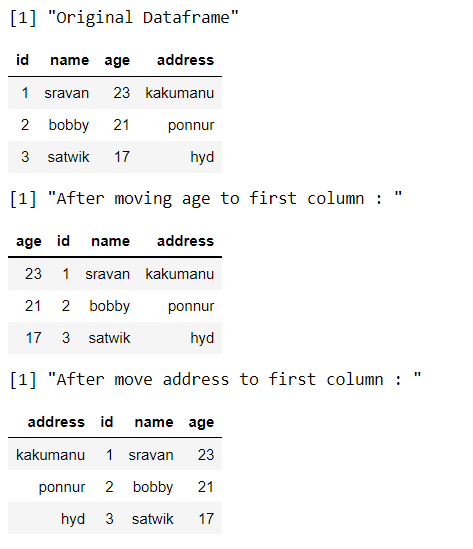Related Articles

# Move Column to First Position of DataFrame in R

• Last Updated : 23 Aug, 2021

In this article, we are going to see how to move particular column in the dataframe to the first position of the dataframe in R Programming language.

Create dataframe for demonstration:

## R

 `# create a dataframe with 4 columns``# they are id,name,age and address``data = ``data.frame``(id=``c``(1, 2, 3),``                  ``name=``c``(``"sravan"``, ``"bobby"``,``                         ``"satwik"``),``                  ``age=``c``(23, 21, 17),``                  ``address=``c``(``"kakumanu"``, ``"ponnur"``, ``"hyd"``))`` ` `# display``data`

Output:## Method 1: Using Base R

In this method we will move the columns to the first position using base R language.

Syntax: dataframe[ , c(“column_name”, names(dataframe)[names(dataframe) != “column_name”])]

where

• dataframe is the input dataframe
• column_name is the name of the column to be shifted to the first

We are going to move a particular column to first position using index operator and by c() function, we will combine the column name and then pushed to first position in the dataframe.

Example: R program to shift the columns to first for the above-created dataframe.

## R

 `# create a dataframe with 4 columns``# they are id,name,age and address`` ` `data = ``data.frame``(id = ``c``(1,2,3),``                  ``name = ``c``(``"sravan"``,``"bobby"``,``                           ``"satwik"``),``                  ``age = ``c``(23,21,17),``                  ``address = ``c``(``"kakumanu"``,``"ponnur"``,``"hyd"``))`` ` `# display``print``(``"Original Dataframe"``)``data`` ` `print``(``"After moving age to first column : "``)`` ` `# move age to first column``data_Sorted = data[ , ``c``(``"age"``,``                        ``names``(data)[``names``(data) != ``"age"``])]`` ` `# display sorted data``data_Sorted`` ` `print``(``"After move address to first column : "``)`` ` `# move address to first column``data_Sorted1 = data[ , ``c``(``"address"``, ``                         ``names``(data)[``names``(data) != ``"address"``])]`` ` `# display sorted data``data_Sorted1`

Output:## Method 2 : Using dplyr() package

By using this package we can shift particular column to the first, Here we are using %>% operator to load the shifted data into dataframe and use the select () function that takes the particular column name to be shifted and every() thing is used to get the dataframe data

Syntax: dataframe%>% dplyr::select(“column_name”, everything())

where

• dataframe is the input dataframe
• column_name is the column to be shifted to first

Example: R program to shift particular column to first

## R

 `# load the dplyr package``library``(``"dplyr"``)`` ` `# create a dataframe with 4 columns``# they are id,name,age and address`` ` `data = ``data.frame``(id = ``c``(1,2,3),``                  ``name = ``c``(``"sravan"``,``"bobby"``,``                           ``"satwik"``),``                  ``age = ``c``(23,21,17),``                  ``address = ``c``(``"kakumanu"``,``"ponnur"``,``"hyd"``))`` ` `# display``print``(``"Original Dataframe"``)``data`` ` `print``(``"After moving age to first column : "``)`` ` `# move age to first column``data_Sorted = data %>% dplyr::``select``(``"age"``, ``                                     ``everything``())`` ` `# display sorted data``data_Sorted`

Output:My Personal Notes arrow_drop_up